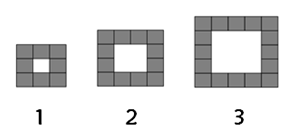## Thursday, 29 March 2018

### PatternsWhat is the rule for this pattern?
What would the next two numbers in the pattern be?
20, 24
What would the 100th number be?
400

Complete the table if the rule is: 5N-3

 Nth term (pattern number) 1 2 3 Number of blocks 2 4 6

### 3D shapes

This is a cuboid it has 2 square faces and 4 rectangle faces. It also has 11 vertices and 8 edges. Can you name 4 cuboids that you know? Where can you find a cuboid?

This is a cylinder it has 2 circle faces no edges and no vertices Can you find some cylinders in your classroom or house? What are some cylinders you know?

## Thursday, 22 March 2018

### Spectacular shapes Level 2 learning

Spectacular Shapes
We are learning to identify shapes by their properties

Algebra Recap:

 6A = 36  A =30 6B = 48  B = 42 14 - C = 5.  C = 9 20 + D = 29. D =9 E - 6 = 15. E = 9 F x 2 + 1 = 21. F = 10 5G = 30. G =25 12H + 2 = 62. H = 58

Name the following shapes:Name the following shapes:Identify the following shapes based on their properties:

 Name of the Shape Properties parallelogram A Quadrilateral with four even sides and four vertices with 90 degree angles octagon A Polygon with 8 sides Isosceles triangle A three sided polygon with two sides that are the same length trapezoid A quadrilateral with one set of parallel sides cube A 3D shape with all sides of equal length and 6 faces Square prism A 3D shape with 6 faces; 2 square shaped faces and 4 rectangle shaped faces sphere A 3D shape with no sides or edges

## Friday, 16 March 2018

### Understanding Shapes

Walt: describe shapes by there properties.This is a triangular prism it has:
2 Triangular faces
3 Rectangular Faces
and 9 vertices

This is a square prism it has:
2 Square faces4 Rectangular faces
and 11 Vertices

Task Description: For this task I was given pipe cleaners to make 3D shapes so I decided to Make a Triangular prism and a Square prism. I then had to identify the faces and vertices. I put it onto my blog with a Title, Labels, Task Description, and a Walt.

## Wednesday, 7 March 2018

### What is a function?

A function is a group of group of commands used to make one command, for example tying your shoelace is a function and doing your collar is a function. Our life is full of functions. Functions are used in things like dances books and even going to the toilet. Functions are often used in programs and code all around the world. The main definition of a function is; a relation or expression involving one or more variables.